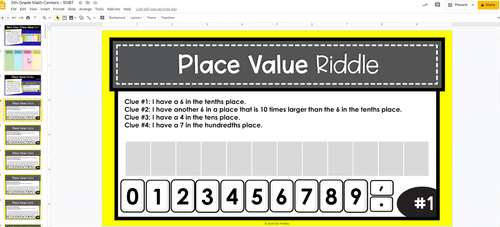# 5th Grade Math Centers - Base Ten with Digital Math Centers - Distance Learning

Rated 4.87 out of 5, based on 409 reviews
409 Ratings;
5th
Subjects
Resource Type
Standards
Formats Included
• PDF
•Google Apps™
Pages
150 + Digital Math Centers
Report this resource to TPTThe Teacher-Author indicated this resource includes assets from Google Workspace (e.g. docs, slides, etc.).

#### What educators are saying

A variety of activities are available and Jennifer Findley's work is always high quality. I never hesitate to use any resources she puts on TPT.
I use these for fluency review after teaching the skill. This is an awesome resource for hands on and engaging learning.
##### Also included in
1. 5th Grade Math Centers for Every Standard! Each standard has several math centers to allow you to differentiate and use this bundle ALL YEAR! There are over 150 math centers for 5th grade in this huge bundle!Need another grade level?Click here to see 3rd Grade Math Centers.Click here to see 4th Grad
Price \$38.00Original Price \$58.00Save \$20.00
2. Do you want your math stations and guided math centers done for the year? This 5th grade guided math bundle is the only resource you will need to have your math centers done for the year!Need Another Grade Level?Click here to see the 3rd Grade Guided Math Mega Bundle.Click here to see the 4th Grade
Price \$95.00Original Price \$176.50Save \$81.50

### Description

Need 5th grade math centers that are rigorous and engaging? This fifth grade math center resource includes 22 math centers (digital AND printable) that review the Number and Operations: Base Ten Math Standards for 5th grade.

Note: This resource now includes BOTH printable and digital math centers! See pages 4-11 for the details on the digital math centers.

•Each center is available in black and white only, to save ink. I printed these centers in colored card stock to add some color.

•Each center includes a teacher notes page with a picture of the center in action, a student directions page, the center cards or pieces, recording sheets, and an answer key when applicable. The nature of some of the centers don’t allow for answer keys.

•The only manipulatives needed for this center are dice and a paperclip to create a spinner.

Here are the names/skills for the printable 5th grade math centers:

• Next Door Place Value Sort
• Place Value Riddles
• Working with the Powers of 10
• Rolling and Spinning with Place Value
• Representing Decimals Go Fish
• Number Round Up
• Decimal Memory
• Decimal Puzzles
• Decimal War
• True or False
• Roll and Round
• Spin and Round
• Rounding and Comparing True or False Sort
• Multiplication Puzzles
• Roll and Spin a Division Problem
• Division Remainder Sort
• Multiplication and Division Word Problems
• Decimal Sums and Differences
• Decimal Operations Puzzles
• Printable Decimal Operation Games
• Decimal Word Problems

•Each digital math center includes a direction page with a picture of the center.

•Recording sheets are not included since the students do all of the work on the slides.

*The content for the digital math centers is the SAME as the printable math centers. It has been modified for optimal digital use and engagement.

Here are the names/skills for the 5th grade digital math centers (REMEMBER THAT THE CONTENT IS THE SAME AS THE PRINTABLE MATH CENTERS):

• Next Door Place Value Sort
• Place Value Riddles Version A
• Place Value Riddles Version B
• Multiplying and Dividing by Powers of 10 – Version A – Exponents
• Multiplying and Dividing by Powers of 10 – Version B – No Exponents
• Number Form Round Up - Writing and Representing Decimals
• Decimal Match - Writing and Representing Decimals
• Decimal War - Comparing Decimals
• True or False - Comparing Decimals
• Drag and Round
• Spin and Round
• Rounding and Comparing True or False Sort
• Multiply Madness - Multi-Digit Multiplication
• Multiplication Match - Multi-Digit Multiplication
• Drag and Drop Division 2-Digit Divisors
• Drag and Drop Division 1 -Digit Divisors
• Division Remainder Sort
• Multiplication and Division Word Problems
• Decimal Sums and Differences with Models Version A
• Decimal Sums and Differences with Models Version B
• Decimal Operations Puzzles
• Decimal Operations Review
• Decimal Word Problems

More 5th Grade Math Centers and Activities

Total Pages
150 + Digital Math Centers
Included
Teaching Duration
N/A
Report this resource to TPT
Reported resources will be reviewed by our team. Report this resource to let us know if this resource violates TPT’s content guidelines.

### Standards

to see state-specific standards (only available in the US).
Recognize that in a multi-digit number, a digit in one place represents 10 times as much as it represents in the place to its right and 1/10 of what it represents in the place to its left.
Explain patterns in the number of zeros of the product when multiplying a number by powers of 10, and explain patterns in the placement of the decimal point when a decimal is multiplied or divided by a power of 10. Use whole-number exponents to denote powers of 10.
Read, write, and compare decimals to thousandths.
Use place value understanding to round decimals to any place.
Fluently multiply multi-digit whole numbers using the standard algorithm.